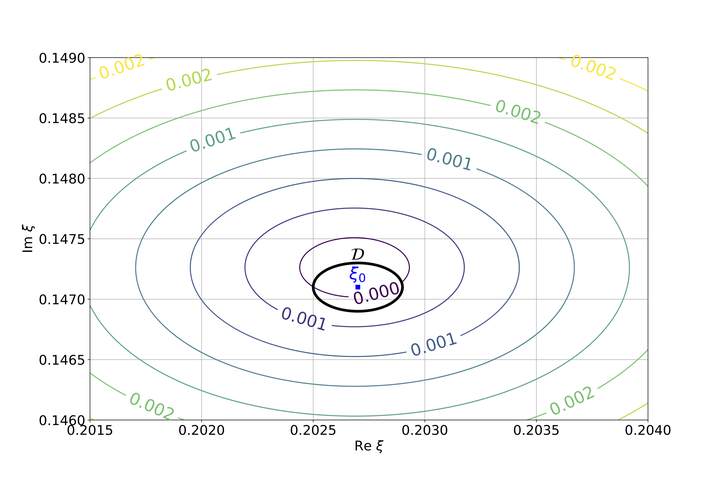# A stiffly stable semi-discrete scheme for the characteristic linear hyperbolic relaxation with boundary### Résumé

We study the stability of the semi-discrete central scheme for the linear damped wave equation with boundary. We exhibit a sufficient condition on the boundary to guarantee the uniform stability of the initial boundary value problem for the relaxation system independently of the stiffness of the source term and of the space step. The boundary is approximated using a summation-by-parts method and the stiff stability is proved using energy estimates and the Laplace transform. We also investigate if the condition is also necessary, following the continuous case studied by Xin and Xu (J. Differential Equations 2000).

Type
Publication
ESAIM: Mathematical Modelling and Numerical Analysis